Successfully reported this slideshow.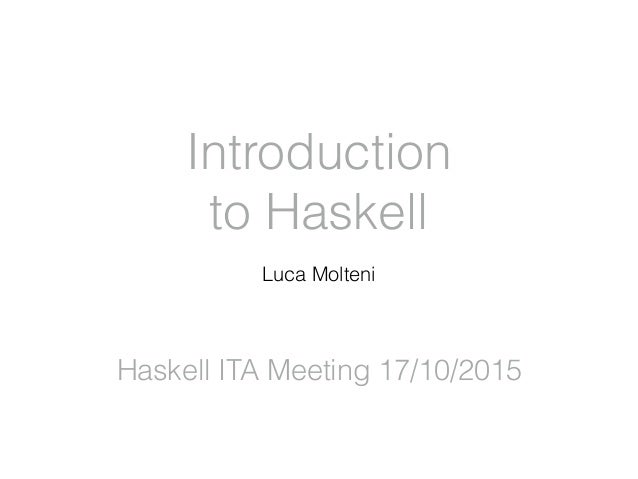Upcoming SlideShare
×

of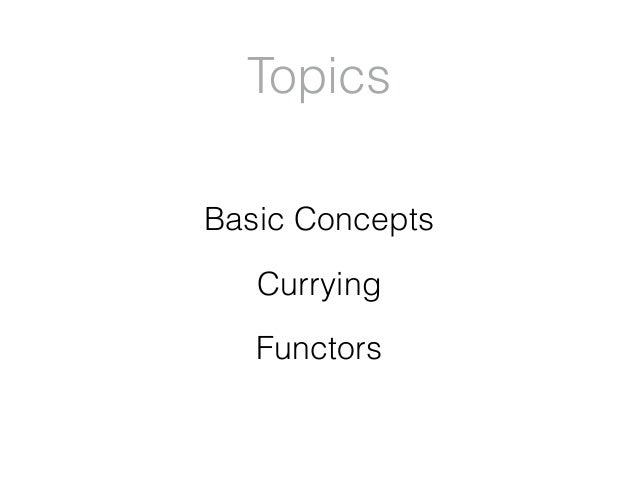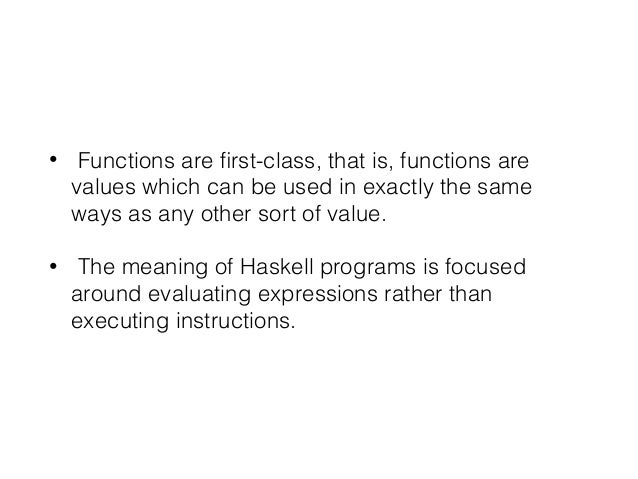Upcoming SlideShare
What is Pure Functional Programming, and how it can improve our application testing?
Next

3

Share

A simple introduction to Haskell during the 17/10/2015 Haskell ITA meetup in Bologna.
It shows basic Haskell concepts, currying and functors.

See all

### Related Audiobooks

#### Free with a 30 day trial from Scribd

See all

2. 2. Topics Basic Concepts Currying Functors
3. 3. • Functions are ﬁrst-class, that is, functions are values which can be used in exactly the same ways as any other sort of value. • The meaning of Haskell programs is focused around evaluating expressions rather than executing instructions.
4. 4. 3 * (9 + 5) => 3 * 14 => 42
5. 5. 3 * (9 + 5) => 3 * 9 + 3 * 5 => 27 + 3 * 5 => 27 + 15 => 42
6. 6. simple x y z = x * (y + z)
7. 7. simple 3 9 5 => 3 * (9 + 5) => 3 * 14 => 42
8. 8. “An expression is said to be referentially transparent if it can be replaced with its value without changing the behavior of a program (in other words, yielding a program that has the same effects and output on the same input).” Referential transparency
9. 9. simple a b c = simple a c b
10. 10. simple a b c => { unfold } a * (b + c) => { commutativity } a * (c + b) => { fold } simple a c b
11. 11. x = x + 1
12. 12. x = x + 1 x => x + 1 => (x + 1) + 1 => ((x + 1) + 1) +1 => (((x + 1) +1) +1) +1 ...
13. 13. “Because a referentially transparent expression can be evaluated at any time, it is not necessary to deﬁne sequence points nor any guarantee of the order of evaluation at all. Programming done without these considerations is called purely functional programming.” Referential transparency
14. 14. totalArea r1 r2 r3 = pi * r1 ^ 2 + pi * r2 ^ 2 + pi * r3 ^ 2 Sum of the areas of three circles with radii r1, r2, r3
15. 15. circleArea r = pi * r ^ 2 totalArea r1 r2 r3 = circleArea r1 + circleArea r2 + circleArea r3 Sum of the areas of three circles with radii r1, r2, r3
16. 16. r1 = 3 r2 = 4 r3 = 5 radii = r1 : r2 : r3 : [] Sum of the areas of n circles?
17. 17. radii :: [Float] radii = r1 : r2 : r3 : [] Sum of the areas of n circles?
18. 18. List data List a = [] | a : List a
19. 19. totalArea :: [Float] -> Float totalArea [] = 0 Sum of the areas of three circles with radii r1, r2, r3
20. 20. totalArea :: [Float] -> Float totalArea [] = 0 totalArea (x : xs) = circleArea x + totalArea xs Sum of the areas of three circles with radii r1, r2, r3
21. 21. square :: [Int] -> [Int] square [] = [] square (x : xs) = (x ^ 2) : square xs Square of list
22. 22. Map map :: (a -> b) -> [a] -> [b]
23. 23. totalArea :: [Float] -> [Float] totalArea = map circleArea Sum of the areas of three circles with radii r1, r2, r3
24. 24. totalArea :: [Float] -> Float totalArea = sum . map circleArea Sum of the areas of three circles with radii r1, r2, r3
25. 25. Currying
26. 26. simple :: (Int -> Int -> Int) -> Int simple (x y z) Currying
27. 27. simple :: Int -> Int -> Int -> Int simple x y z = x * ( y + z) (((simple x) y) z) Currying
28. 28. simple 5 Partial Application
29. 29. simple 5 :: Int -> Int -> Int simple 5 Partial Application
30. 30. simple :: Int -> Int -> Int -> Int simple 5 :: Int -> Int -> Int simple 5 2 :: Int -> Int simple 5 2 3 :: Int Partial Application
31. 31. Point free programming Tacit programming (point-free programming) is a programming paradigm in which a function deﬁnition does not include information regarding its arguments, using combinators and function composition [...] instead of variables.
32. 32. Point free programming map (x -> increment x) [2,3,4] [3,4,5] map increment [2,3,4] [3,4,5] map (x -> x + 1) [2,3,4] [3,4,5] map (+1) [2,3,4] [3,4,5] increment :: Int -> Int increment x = x + 1
33. 33. Point free programming mf criteria operator list = filter criteria (map operator list) mf = (. map) . (.) . filter
34. 34. Functors
35. 35. Functors map :: (a -> b) -> [a] -> [b] data List a = [] | a : List a
36. 36. Functors treeMap :: (a -> b) -> Tree a -> Tree b data Tree a = Leaf a | Branch (Tree a) (Tree a)
37. 37. Functors treeMap :: (a -> b) -> Tree a -> Tree b map :: (a -> b) -> [a] -> [b]
38. 38. Functors thingMap :: (a -> b) -> f a -> f b
39. 39. Functors A typeclass is a class of types that behave similarly.
40. 40. Functors class Functor f where fmap :: (a -> b) -> f a -> f b
41. 41. Functors instance Functor [] where fmap = map
42. 42. Functors / Maybe data Maybe a = Just a | Nothing
43. 43. Functors instance Functor Maybe where fmap _ Nothing = Nothing fmap f (Just a) = Just (f a)
44. 44. Functors / Maybe fmap (* 2) (Just 2) Just 4 fmap (+ 5) (Just 2) Just 7 fmap (+ 5) (Nothing) Nothing
45. 45. The End @volothamp It’s not about the destination. It’s about the journey.
•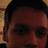#### LuigiCandita

Oct. 3, 2018
•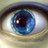#### ivanopagano

Mar. 4, 2016
•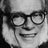#### lucazulian90

Oct. 17, 2015

Total views

2,952

On Slideshare

0

From embeds

0

Number of embeds

251

53

Shares

0